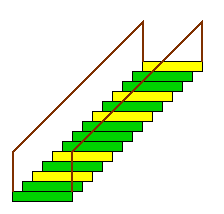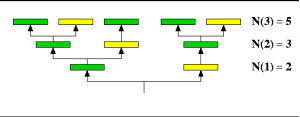# Ways to paint stairs with two colors such that two adjacent are not yellow

Given n stairs and we have 2 colour yellow and green the task is that we have to paint given stairs by given colour with condition is that we cannot paints two yellow steps directly after each other.

Examples :

```Input : n = 1
Output : 2
A single stair can be colored either
as green or yellow.

Input : n = 3
Output : 5
```Case 1: When we have 1 stair, we can paint either yellow or green.
Case 2: When we have 2 stairs, we can paint first stair by either yellow or green but for next stair we can only paint by green because we cannot paint two yellow steps directly after each other. So total cases are three YG, GG, GY.
Case 3: When we have 3 stairs then we can paint it by in 5 ways.

If we take a closer look, we can notice that it follows Fibonacci Series.## C++

 `// C++ Program to find the number of ways to paint stairs ` `#include ` `using` `namespace` `std; ` ` `  `// Function to find the number of ways ` `int` `ways(``int` `n) ` `{ ` `    ``int` `W[n + 1]; ` ` `  `    ``// take base case for 1 and 2 ` `    ``W = 2; ` `    ``W = 3; ` ` `  `    ``for` `(``int` `i = 3; i <= n; i++)  ` `        ``W[i] = W[i - 1] + W[i - 2]; ` `     `  `    ``return` `W[n]; ` `} ` ` `  `// Driven code ` `int` `main() ` `{ ` `    ``int` `n = 3; ` `    ``printf``(``"%d"``, ways(n)); ` `    ``return` `0; ` `} `

## Java

 `// java Program to find the number of ` `// ways to paint stairs ` `import` `java.io.*; ` ` `  `public` `class` `GFG { ` `     `  `    ``// Function to find the number of ways ` `    ``static` `int` `ways(``int` `n) ` `    ``{ ` `        ``int` `[]W = ``new` `int``[n+``1``]; ` `     `  `        ``// take base case for 1 and 2 ` `        ``W[``1``] = ``2``; ` `        ``W[``2``] = ``3``; ` `     `  `        ``for` `(``int` `i = ``3``; i <= n; i++)  ` `            ``W[i] = W[i - ``1``] + W[i - ``2``]; ` `         `  `        ``return` `W[n]; ` `    ``} ` `     `  `    ``// Driven code ` `    ``static` `public` `void` `main (String[] args) ` `    ``{ ` `        ``int` `n = ``3``; ` `         `  `        ``System.out.println(ways(n)); ` `    ``} ` `} ` ` `  `// This code is contributed by vt_m. `

## Python3

 `# Python3 code to find the number ` `# of ways to paint stairs ` ` `  `# Function to find the number of ways ` `def` `ways( n ): ` `    ``W ``=` `list``() ` `     `  `    ``# take base case for 1 and 2 ` `    ``W.append(``0``) ` `    ``W.append(``2``) ` `    ``W.append(``3``) ` `     `  `    ``i ``=` `3` `    ``while` `i <``=` `n: ` `        ``W.append(W[i ``-` `1``] ``+` `W[i ``-` `2``]) ` `        ``i ``=` `i ``+` `1` `         `  `    ``return` `W[n] ` ` `  `# Driver code ` `n ``=` `3` `print``(ways(n)) ` ` `  `# This code is contributed by "Sharad_Bhardwaj". `

## C#

 `// C# Program to find the number of ` `// ways to paint stairs ` `using` `System; ` ` `  `public` `class` `GFG { ` `     `  `    ``// Function to find the number of ways ` `    ``static` `int` `ways(``int` `n) ` `    ``{ ` `        ``int` `[]W =``new` `int``[n+1]; ` `     `  `        ``// take base case for 1 and 2 ` `        ``W = 2; ` `        ``W = 3; ` `     `  `        ``for` `(``int` `i = 3; i <= n; i++)  ` `            ``W[i] = W[i - 1] + W[i - 2]; ` `         `  `        ``return` `W[n]; ` `    ``} ` `     `  `    ``// Driven code ` `    ``static` `public` `void` `Main () ` `    ``{ ` `        ``int` `n = 3; ` `     `  `        ``Console.WriteLine(ways(n)); ` `    ``} ` `} ` ` `  `// This code is contributed by vt_m. `

## PHP

 ` `

Output :

```5
```

Time Complexity : O(n)
Extra Space : O(n)

We can solve this problem in O(Log n) time also using matrix exponentiation solution for n-th Fibonacci Number.

My Personal Notes arrow_drop_upCheck out this Author's contributed articles.

If you like GeeksforGeeks and would like to contribute, you can also write an article using contribute.geeksforgeeks.org or mail your article to contribute@geeksforgeeks.org. See your article appearing on the GeeksforGeeks main page and help other Geeks.

Please Improve this article if you find anything incorrect by clicking on the "Improve Article" button below.

Improved By : vt_m, jit_t, PKumar7

Article Tags :

5

Please write to us at contribute@geeksforgeeks.org to report any issue with the above content.